﻿ R 语言的高颜值的配图法则 | 论文写作 - 婷婷旅游课堂# R 语言的高颜值的配图法则 | 论文写作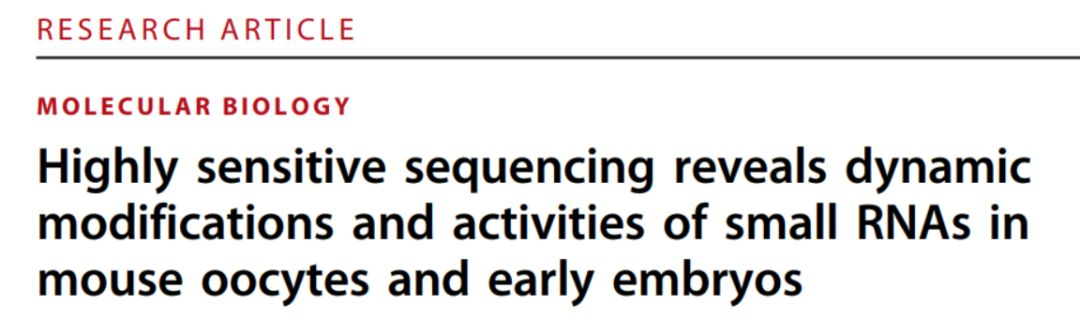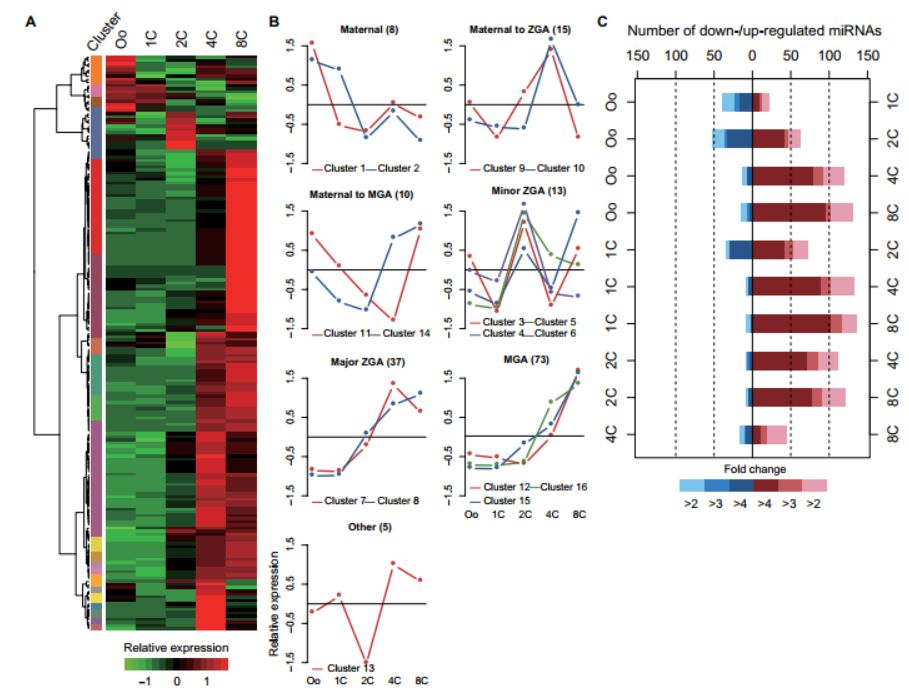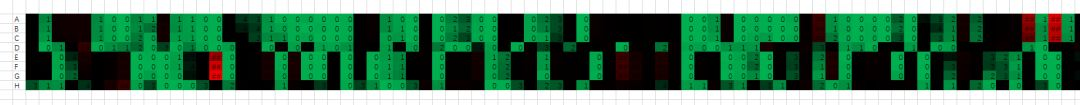What？这完全没有办法聚类好么，我还怎么看差异变化？把这样的热图给老板，别说文章凉了，能不能走出老板办公室都是问题。1 基本图绘制

> setwd("D:/workdir/")  #设置工作目录

>install.packages(“ggplot2”)  #安装 pheatmap 程序包

> library(ggplot2)  #加载 ggplot2 程序包

> data <- read.csv("text.csv",header = T,row.names = 1) #读取数据，读取 csv 格式数据，header = T 代表首行为表头，row.names = 1 代表首列为行名。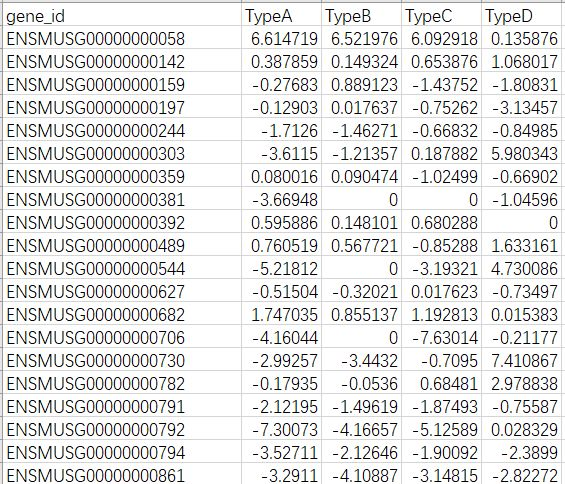（1）散点图

> p <- ggplot(data,aes(x=data\$TypeA,y=data\$TypeB)) + geom_point() # data 代表数据名，aes(x=data\$TypeA,y=data\$TypeB) 代表横坐标与纵坐标，geom_point() 可以表示绘制散点

> p #展示结果图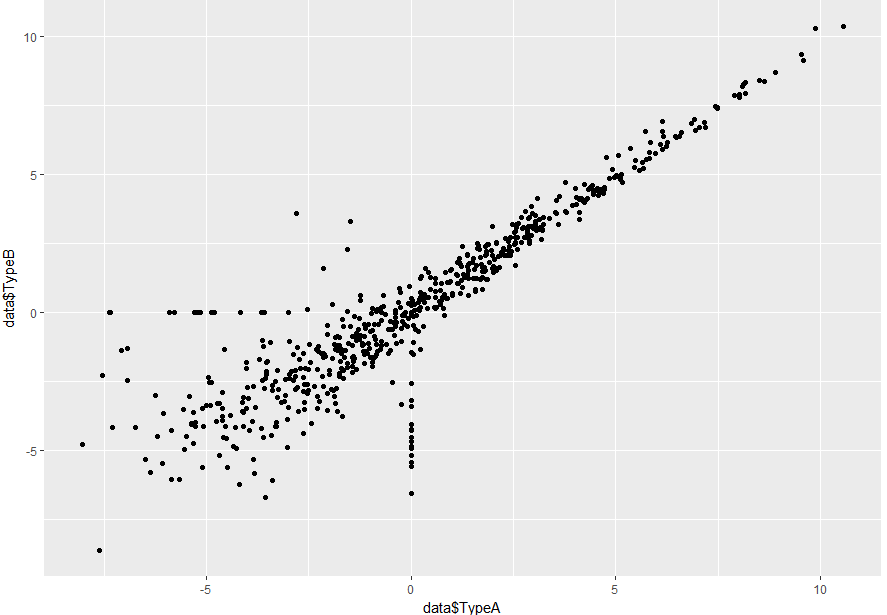（2）折线图

> p <- ggplot(data,aes(x=data\$TypeA,y=data\$TypeB)) + geom_line() + geom_point()  # geom_line() 代表绘制折线，geom_point() 存在与否可以表示点的标注。

> p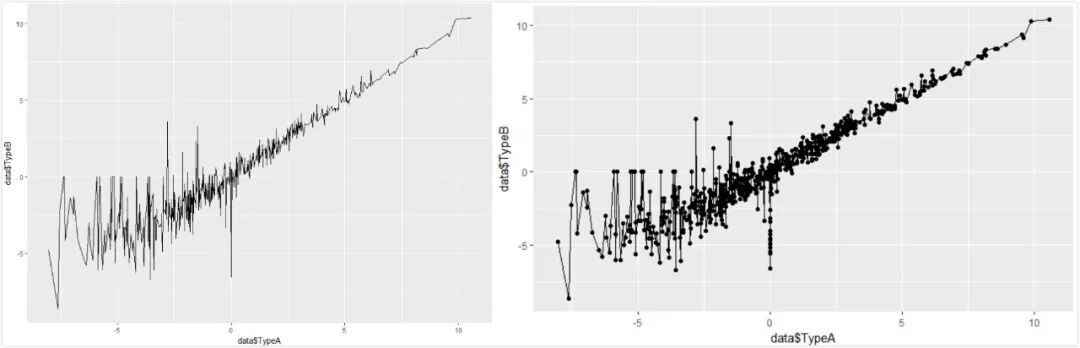（3）条形图 （为了更直观，笔者截取了部分数据

> p <- ggplot(data,aes(x=data\$gene_id,y=data\$TypeB)) + geom_bar(stat = "identity") # geom_bar() 代表绘制直方图。

> p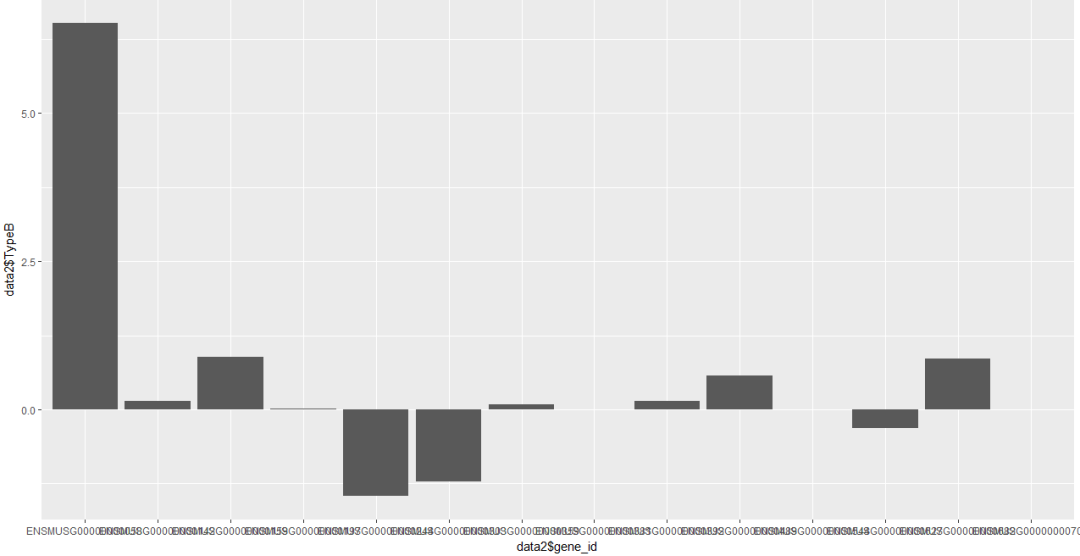（4）箱线图

> p <- ggplot(data3,aes(x=data3\$Type,y=data3\$data)) + geom_boxplot() # geom_boxplot() 代表绘制箱线图。

> p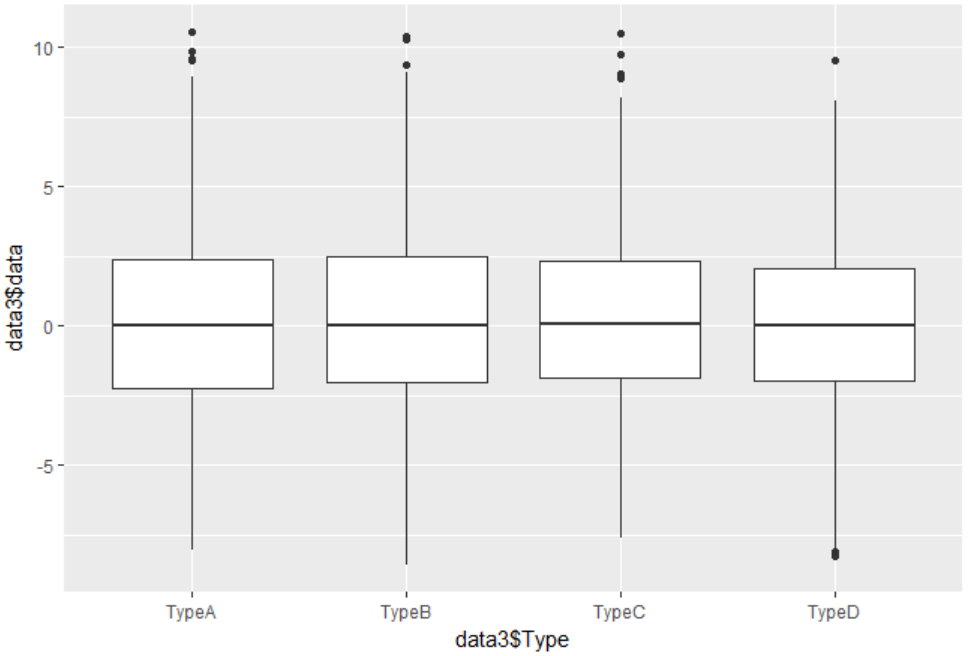（5）小提琴图

> p <- ggplot(data3,aes(x=data3\$Type,y=data3\$data)) + geom_violin() # geom_violin() 代表绘制小提琴图。

> p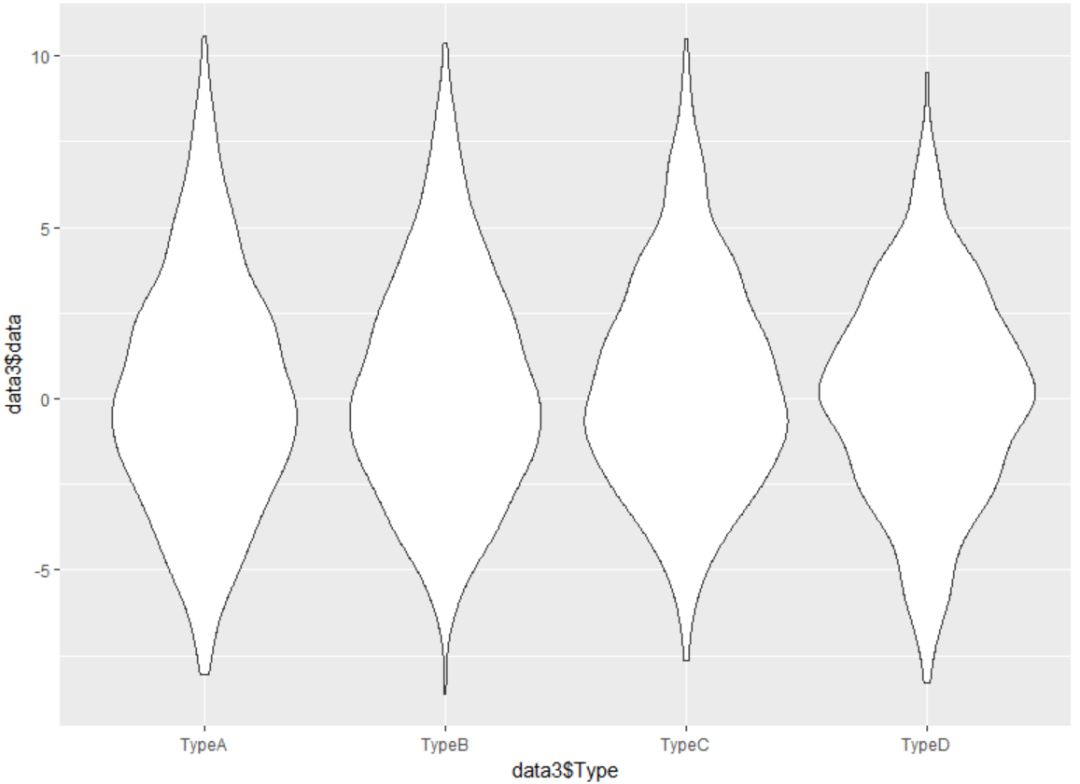2 基本图修饰

（1）配色

> display.brewer.all() #用这个命令可以生成一张图查看本程序的调色板，如下图所示：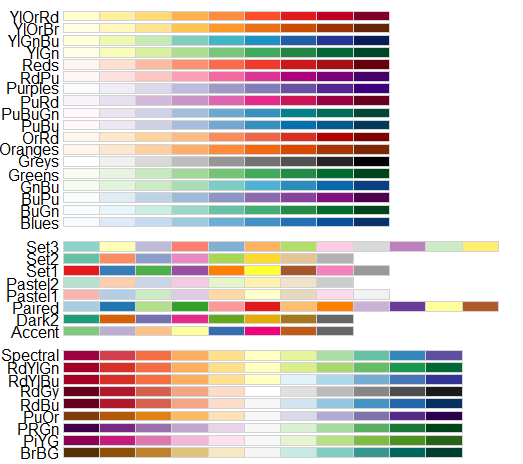> p <- ggplot(data1,aes(x=data1\$number1,y=data1\$stage2,color=data1\$stage,shape=data1\$value)) +scale_color_brewer(palette = "Blues") +geom_point()# color=data1\$stage 将颜色属性传递给 stage 这一列，shape=data1\$value 将形状属性传递给 value 这一列，scale_color_brewer(palette = "Blues") 使用上图中 Blues 调色板。

> p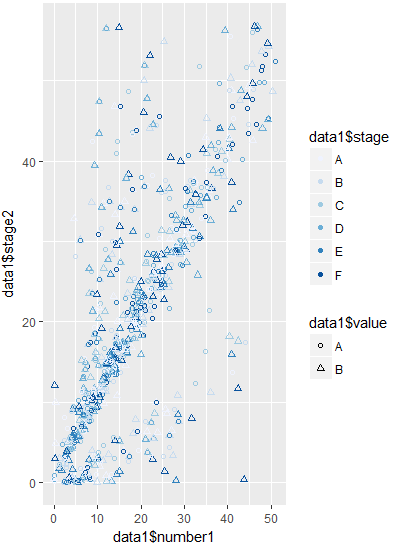（2）坐标轴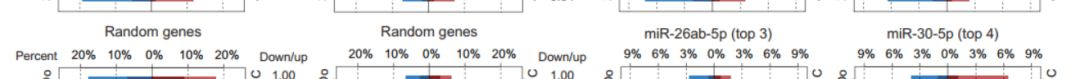> p + coord_flip() #交换 x 轴和 y 轴

> p + ylim(0,15) #如果想截取某一段可以用此命令设置值域。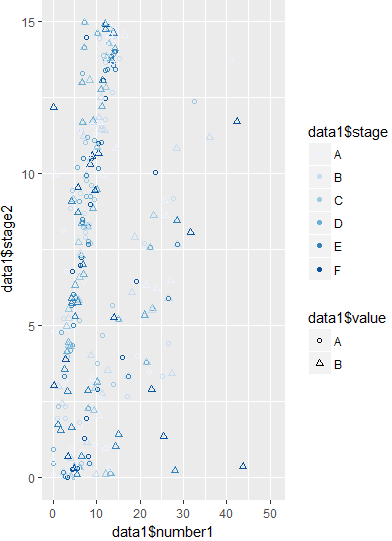> p  + scale_x_continuous(breaks =seq(18,34,50) ) #设置刻度线位置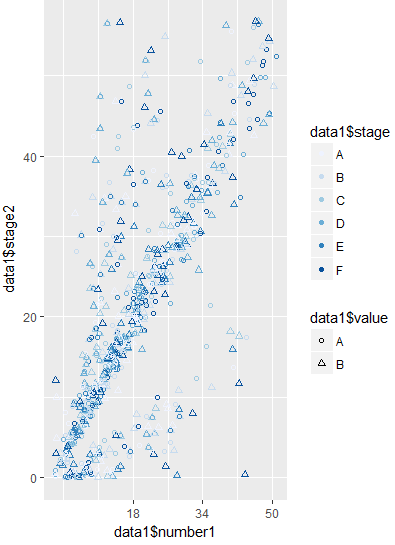> p + theme(axis.text.x = element_text(angle = 90,family = "Times",face = "italic",colour = "darkred",size=rel(0.9))) # angle = 90 设置字体角度, family = "Times" 设置字体族, face = "italic" 设置样式, colour = "darkred" 设置颜色, size=rel(0.9) 设置大小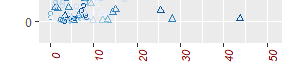> p + xlab("Number of data") #修改坐标文本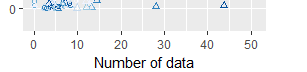（3）误差线修饰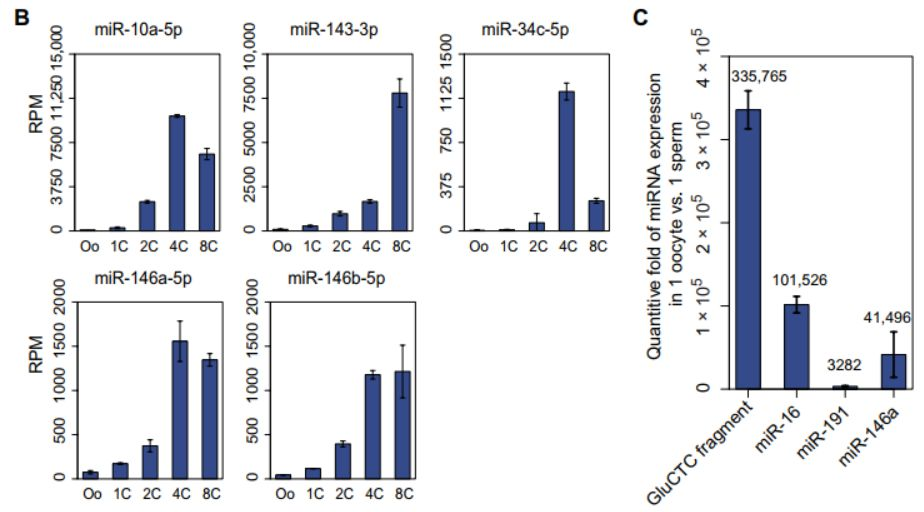p <- ggplot(data1,aes(x=data1\$gene,y=data1\$FPKM,fill=data1\$stage))  #读取数据

+ geom_bar(position = "dodge", stat = "identity") #设置条形图 [position = "dodge"] 代表条形图成簇状分布

+ geom_errorbar(aes(ymin=data1\$FPKM-data1\$Standard.Deviation, ymax=data1\$FPKM+data1\$Standard.Deviation), width=.5,position=position_dodge(.9))  #增加误差线，误差线高度为 FPKM ±Standard.Deviation 两列数据都是提前计算好的。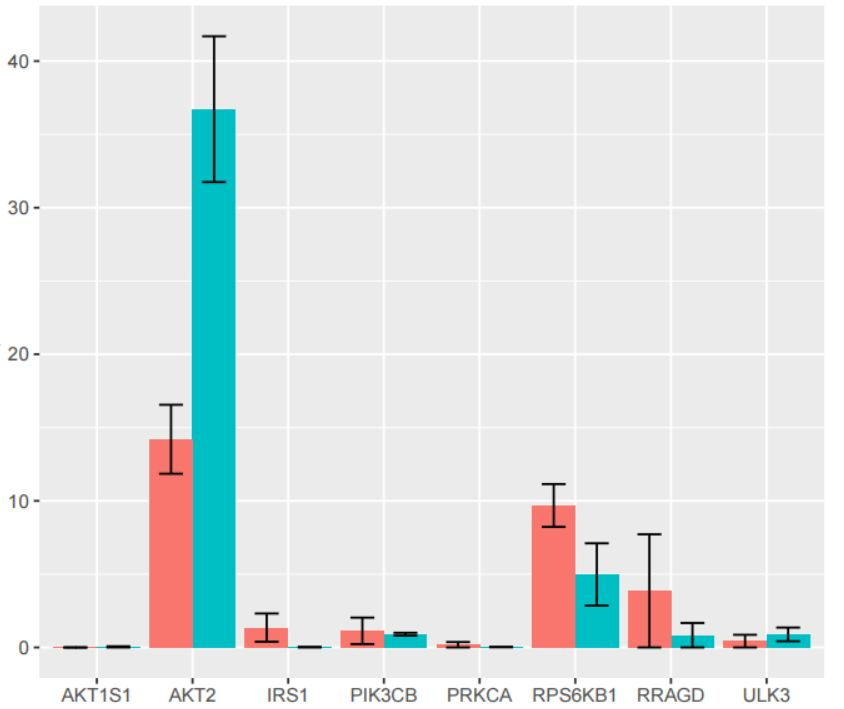（4）基本属性扩展

> p <- ggplot(heightweight,aes(x=ageYear,y=heightIn,colour=sex)) + geom_point()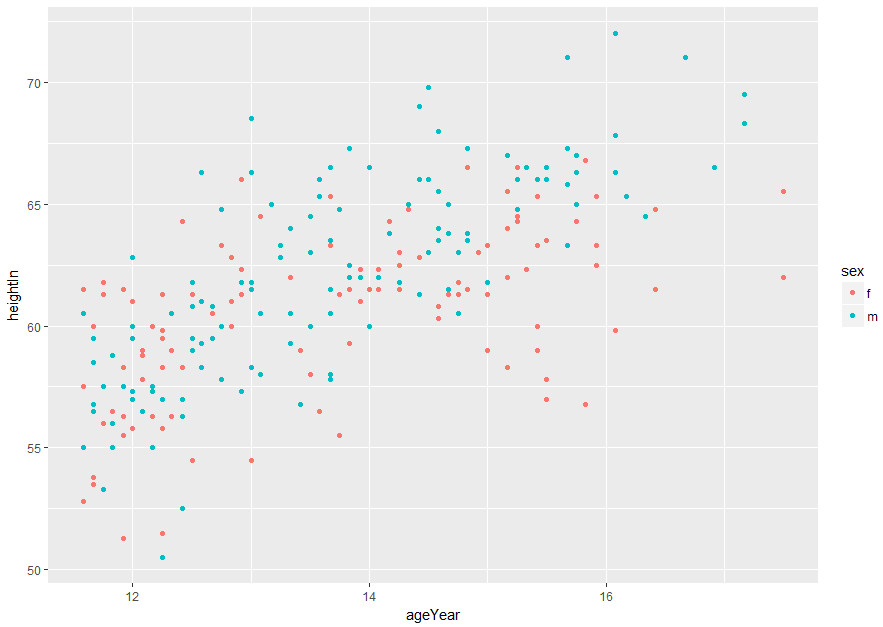①绘图区域选项

> p + theme(panel.grid.major = element_line("red"),panel.grid.minor = element_line(colour = "red",linetype="dashed",size=0.2),panel.background = element_rect(fill="lightblue"),panel.border = element_rect(colour = "blue",fill=NA,size=2)) # panel.grid.minor = element_line(colour = "red",linetype="dashed",size=0.2) 割线颜色，type，尺寸；panel.background = element_rect(fill="lightblue") 背景颜色，panel.border = element_rect(colour = "blue",fill=NA,size=2)) 外框颜色，尺寸。

②文本项目选项

> p + ggtitle("Plot title here") + theme(axis.title.x = element_text(colour="red",size=14),axis.text.x = element_text(colour="blue"),axis.title.y = element_text(colour ="red",size=14,angle=90),axis.text.y=element_text(colour="blue"),plot.title=element_text(colour="red",size=20,face="bold")) # ggtitle("Plot title here") 标题选项；theme 主题选项，其余为文字选项。

③图例选项

> p + theme(legend.background = element_rect(fill="grey85",colour = "red",size=1),legend.title = element_text(colour = "blue",face="bold",size = 14),legend.text = element_text(colour = "red"),legend.key = element_rect(colour = "blue",size = 0.25))

④分面选项

> p + facet_grid(sex ~ .) + theme(strip.background = element_rect(fill="pink"),strip.text.y = element_text(size = 14,angle = -90,face = "bold"))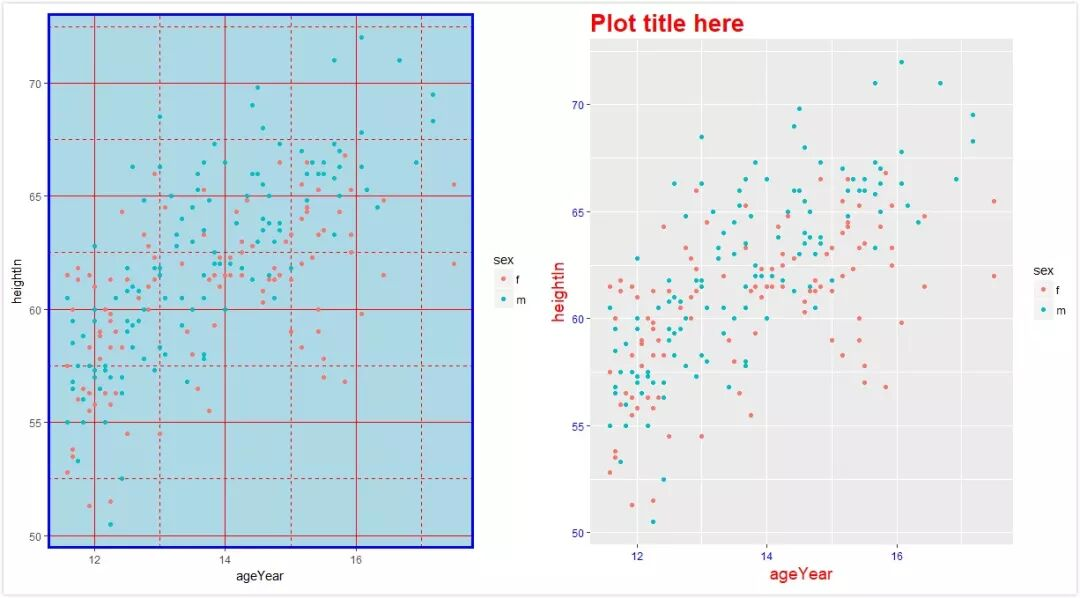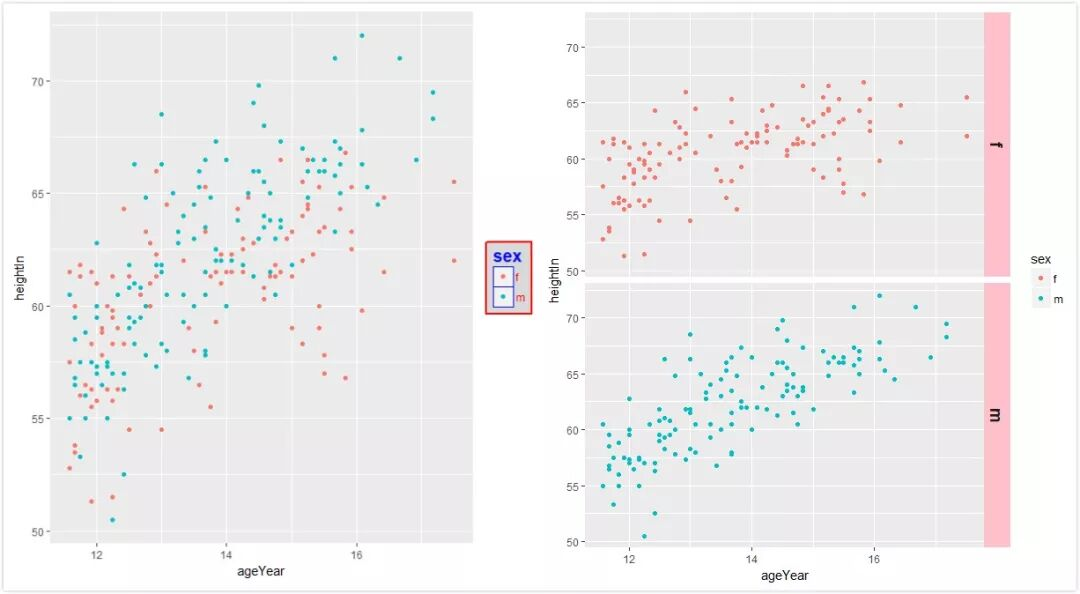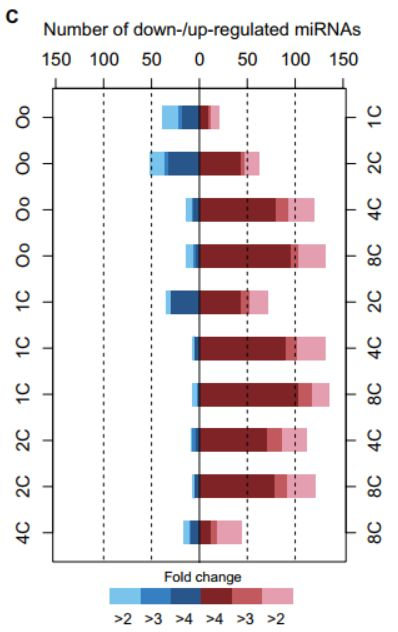>  p <- ggplot(data1,aes(x=data1\$value,y=data1\$number1,fill=data1\$stage))  #写入数据

+geom_bar(stat = "identity",width = 0.6,alpha=.9)  #柱状图基本设置

+ guides(fill=guide_legend(reverse = T))  #图例设置

+coord_flip() #反转坐标

+ theme_bw() + theme(panel.grid.major = element_blank(),panel.grid.minor = element_blank()) + geom_hline(aes(yintercept = 0), data1,alpha=0.2) #设置主题

+ labs(fill="Fold change") +ylab("Picture of text") #坐标标题

+scale_x_discrete(breaks = c("A","B","C","D","E","F"),labels = c("D0","D1,"D2,"D3,"D4,"D5)) #更换刻度文本

+ expand_limits(y=-300) #扩大值域

+ scale_y_continuous(breaks = c(-300,-200,-100,0,100,200,300)) #增加刻度线

+scale_fill_manual (values =c("p"="#3399CC","k"="#996666","4"="#802326","3"="#c2595e","2"="#e49eaf","1.5"="#FFCCCC","-4"="#29568a","-3"="#367fc2","-2"="#79c3ec","-1.5"="#99CCFF")) #更改配色方案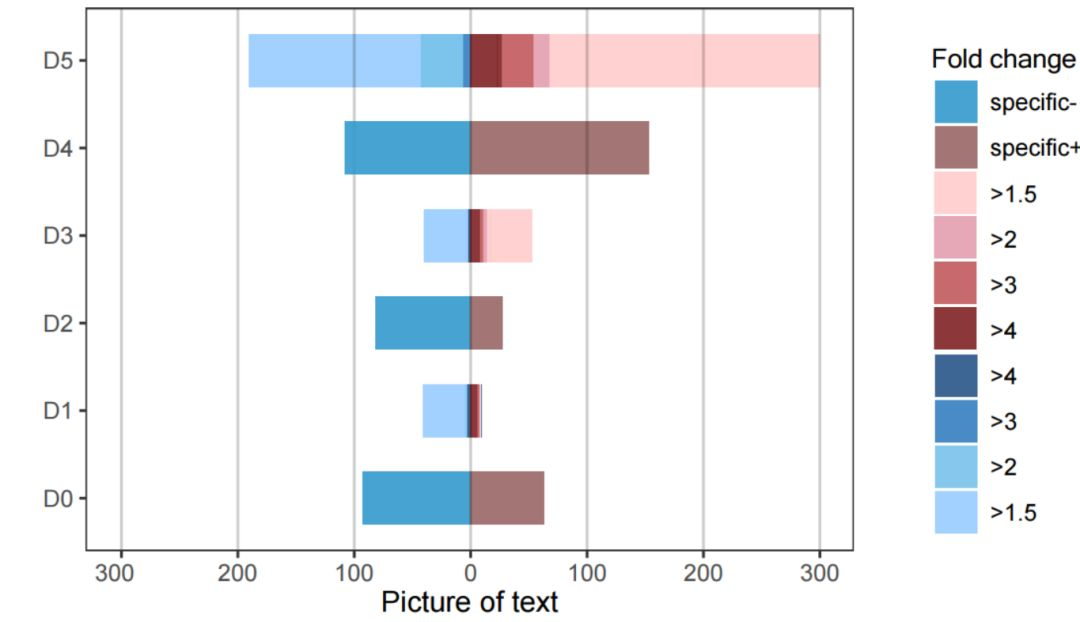3 高级图绘制

（1）基因热图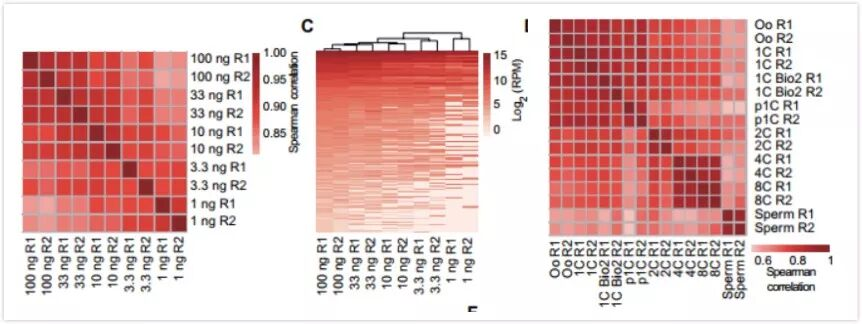>install.packages(“pheatmap”)  #安装 pheatmap 程序包

>library(pheatmap)  #加载 pheatmap 程序包

>setwd("D:/")  #我把文件放在 D 盘，大家根据自己的情况设置

> heatmap.pic1 <- read.csv("text.csv",header = T,row.names = 1)  #文件一般需要 csv 格式，其中 header = T 代表首行为表头，row.names = 1 代表第一列是名字，不做读取。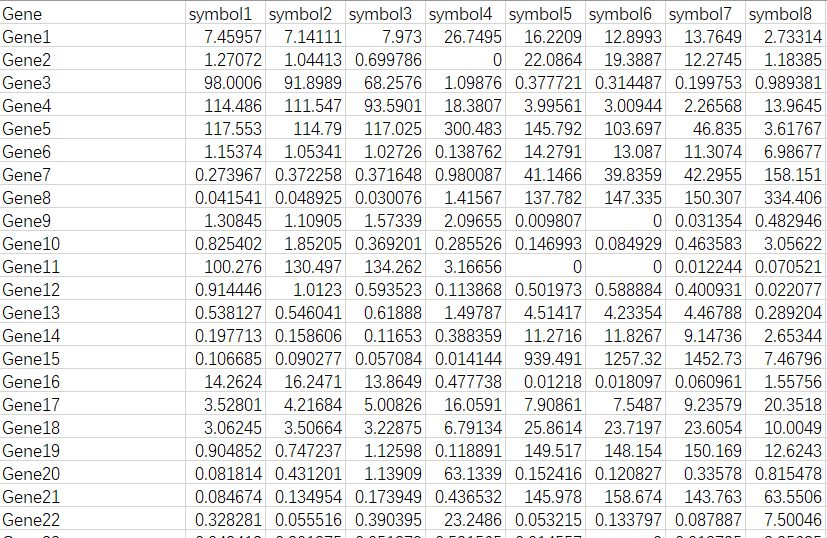pheatmap(heatmap.pic1,  #载入我们的数据

scale="column",  #进行均一化处理, 可以是 "row","column" 以及 "none"，如果不标志这条命令默认是 "none"

clustering_distance_rows = "correlation",  #优化聚类线长度

treeheight_row=25,  #按行聚类树高

treeheight_col=25,  #按列聚类树高

cluster_cols=F,  #是否按列聚类